Explore
Company
Subscribe

# The Mathematics of Gamification

Written by 4SQ ENG on Jan 03, 2014 - Read time: 6 min - Read Later

At Foursquare, we maintain a database of 60 million venues. And like the world it represents, our database is ever-changing, with users from all over the world submitting updates on everything from the hours of a restaurant to the address of a new barbershop. To maintain the accuracy of our venue database, these changes are voted upon by our loyal Superusers (SUs) who vigilantly maintain a watchful eye over our data for their city or neighborhood.

Like many existing crowd-sourced datasets (Quora, Stack Overflow, Amazon Reviews), we assign users points or votes based on their tenure, reputation, and the actions they take. Superusers like points and gamification. It rewards diligent, hard-working SUs (which are the majority) and punishes the few malicious “bad players." But data scientists like probabilities and guarantees. We're interested in making statements like, “we are 99% confident that each entry is correct." How do we allocate points to users in a way that rewards them for behavior but allows us to make guarantees about the accuracy of our database?

At Foursquare, we have a simple, first-principles based method of resolving proposed venue attribute updates. We can gauge each Superuser's voting accuracy based on their performance on honeypots (proposed updates with known answers which are deliberately inserted into the updates queue). Measuring performance and using these probabilities correctly is the key to how we assign points to a Superuser's vote.

The Math

Let's make this more concrete with some math. Let Ho denote the true state of the world, either 1 or -1, which we can interpret as a proposed update being true or false, respectively. We do not observe this but we know Ho= 1 with a-priori probability P0. User 1 votes H1(again, either 1 or -1, representing “yay" or “nay") with independent probability P1 of agreeing with the truth Ho and (1 - P1) of disagreeing. Bayes' Rule then gives us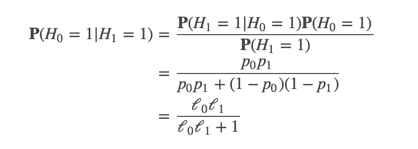where we have written the solution in terms of the likelihood ratio
ℓk=ℓ(pk)ℓk=ℓ(pk) given by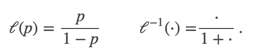Then we have that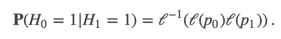In fact, it is easy to see that in the general case,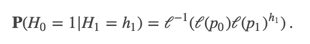Multiplication is hard so we will define the logit or log-likelihood function

logit:(0,1)→(−∞,∞)logit:(0,1)→(−∞,∞)

given by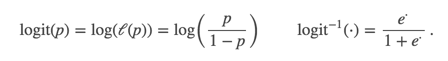Then we have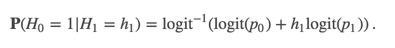Continuing, assume that after user 1 casts their vote, user 2 votes H2 with an independent probability P2 of being correct (i.e. agreeing with Ho). We can think of the posterior probability P(H0=1|H1=h1) as our new prior and inductively repeat the above Bayesian analysis to obtain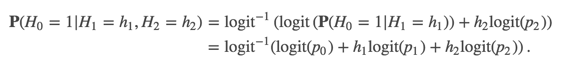In fact, if we have n votes H1,…,Hn, then we have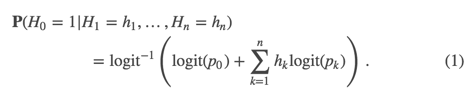The Solution

The above equation suggests that we should assign Sk points or votes to user k based on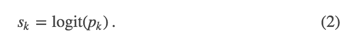We can add up all the “yay" votes and subtract all the “nay" votes to obtain a score for the update. This score can easily be interpreted as a probability that the update is correct. We can set a certainty threshold
pp (e.g. p=99%p=99%) as a threshold for a desired accuracy of this edit. Then, we accept a proposed edit as soon as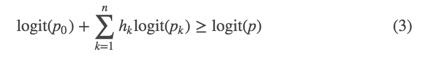In other words, if we take t=logit(p)t=logit(p) to the the points threshold and S0=logit(P0)S0=logit(P0) to be the points allocated to a new proposed edit, then (3) and (4) become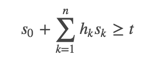and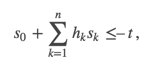which are exactly the equations for voting you would expect. But now, they're derived from math!

The Benefits

• Efficient, data-driven guarantees about database accuracy. By choosing the points based on a user's accuracy, we can intelligently accrue certainty about a proposed update and stop the voting process as soon as the math guarantees the required certainty.
• Still using points, just smart about calculating them. By relating a user's accuracy and the certainty threshold needed to accept a proposed update to an additive point system (2), we can still give a user the points that they like. This also makes it easy to take a system of ad-hoc points and convert it over to a smarter system based on empirical evidence.
• Scalable and easily extensible. The parameters are automatically trained and can adapt to changes in the behavior of the userbase. No more long meetings debating how many points to grant to a narrow use case.
So far, we've taken a very user-centric view of Pk (this is the accuracy of user k). But we can go well beyond that. For example, Pk could be “the accuracy of user k's vote given that they have been to the venue three times before and work nearby." These clauses can be arbitrarily complicated and estimated from a (logistic) regression of the honeypot performance. The point is that these changes will be based on data and not subjective judgments of how many “points" a user or situation should get.

Some practical considerations:

• In practice, we might want a different threshold for accepting (3) versus rejecting (4) a proposed edit.
• For notational simplicity, we have assumed that a false positives and false negatives in user k's voting accuracy have the same probability Pk. In general, this is not the case. We leave it to the reader to figure the math of the general case.
• Users like integer points. We have to round Sk to the nearest integer. Because we can multiply linear equations like (3) and (4) by a positive constant, we can set sk=[α⋅logit(pk)]sk=[α⋅logit(pk)] where [⋅][⋅] is the rounding function and α is a large positive constant. A large α will prevent the loss of fidelity.
• We've explained how to obtain P1,P2,… from honeypots but how do we obtain P0, the accuracy of newly proposed updates. One way is to use the above to bootstrap those accuracies from voting: we can use this voting technique to infer the accuracy of proposals by looking at what fraction of proposed updates are accepted!
• Bayesian Smoothing. We assume a relatively low-accuracy prior for the accuracy of individuals. This is a pessimistic assumption that keeps new, untested users from having too much influence. It also rewards users for lending their judgment and casting votes as long as those are more accurate than our pessimistic prior. Of course, we also increase the likelihood of showing new Super Users honeypots to give them a chance to prove themselves.

— Michael Li
Data Scientist
@tianhuil